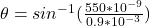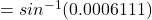## Two narrow parallel slits (separated by the distance d = 0.9 mm) are illuminated by monochromatic light with lambda = 550 nm. The two slits

Question

Two narrow parallel slits (separated by the distance d = 0.9 mm) are illuminated by monochromatic light with lambda = 550 nm. The two slits create a diffraction pattern of bright and dark spots. Find the smallest angle theta1 (in degrees) at which the rays from the two slits create a bright spot.

in progress 0
6 months 2021-07-21T01:59:27+00:00 1 Answers 2 views 0

The smallest angle is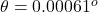Explanation:

From the question we are told that

The distance of separation is  d = 0.9 mm

The wavelength is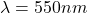General the location of the interference fringes is mathematically represented by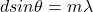m is the order of the fringe

Now the smallest angle at which both ray create a bright spot would be when

m= 1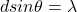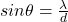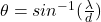Substituting values